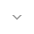belongs to Maven artifact com.android.support:percent:28.0.0-alpha1
Deprecated since version 26.1.0

# PercentLayoutHelper.PercentLayoutInfo

``` public static class PercentLayoutHelper.PercentLayoutInfo ```
``` extends Object ``` ``` ```

 java.lang.Object ↳ android.support.percent.PercentLayoutHelper.PercentLayoutInfo

This class was deprecated in API level 26.1.0.
use ConstraintLayout and Guidelines for layout support.

Container for information about percentage dimensions and margins. It acts as an extension for `LayoutParams`.

## Summary

### Fields

``` public float``` `aspectRatio`

The decimal value of the percentage-based aspect ratio.

``` public float``` `bottomMarginPercent`

The decimal value of the percentage-based bottom margin.

``` public float``` `endMarginPercent`

The decimal value of the percentage-based end margin.

``` public float``` `heightPercent`

The decimal value of the percentage-based height.

``` public float``` `leftMarginPercent`

The decimal value of the percentage-based left margin.

``` public float``` `rightMarginPercent`

The decimal value of the percentage-based right margin.

``` public float``` `startMarginPercent`

The decimal value of the percentage-based start margin.

``` public float``` `topMarginPercent`

The decimal value of the percentage-based top margin.

``` public float``` `widthPercent`

The decimal value of the percentage-based width.

### Public constructors

``` PercentLayoutHelper.PercentLayoutInfo() ```

### Public methods

``` void``` ``` fillLayoutParams(ViewGroup.LayoutParams params, int widthHint, int heightHint) ```

Fills the `width` and `height` fields of the passed `ViewGroup.LayoutParams` object based on currently set percentage values.

``` void``` ``` fillMarginLayoutParams(View view, ViewGroup.MarginLayoutParams params, int widthHint, int heightHint) ```

Fills the margin fields of the passed `ViewGroup.MarginLayoutParams` object based on currently set percentage values and the current layout direction of the passed `View`.

``` void``` ``` fillMarginLayoutParams(ViewGroup.MarginLayoutParams params, int widthHint, int heightHint) ```

This method was deprecated in API level 24.1.0. Use `fillMarginLayoutParams(View, ViewGroup.MarginLayoutParams, int, int)` for proper RTL support.

``` void``` ``` restoreLayoutParams(ViewGroup.LayoutParams params) ```

Restores original dimensions after they were changed for percentage based values.

``` void``` ``` restoreMarginLayoutParams(ViewGroup.MarginLayoutParams params) ```

Restores the original dimensions and margins after they were changed for percentage based values.

``` String``` ``` toString() ```

### Inherited methodsFrom class